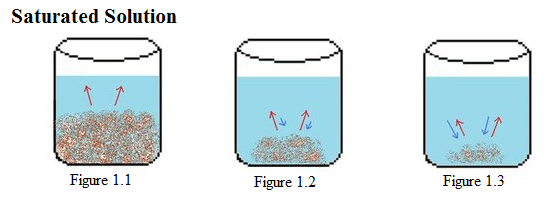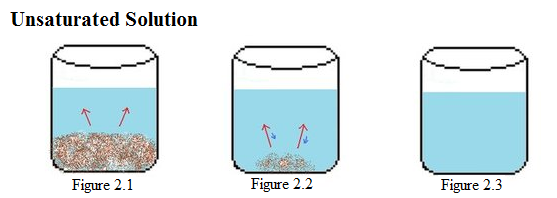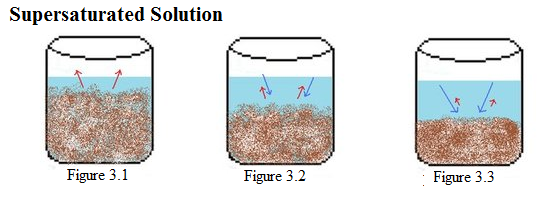Crystal

5-PS1-1

# Saturations - Saturated, Unsaturated, Supersaturated Study Guide

A solution can reach a state of saturation: the point at which a solution of a substance can dissolve no more of that substance! Let's learn more in this guide.

# Introduction

Have you ever made chocolate milk with too much syrup? Some of the extra remains undissolved at the bottom of the glass. That’s because the milk has become a saturated solution, a solution containing the maximum concentration of one or more dissolved solutes in a solvent. In this case, the syrup would be the solute, and the milk the solvent. In a saturated solution, additional solutes won't dissolve (just like the extra chocolate syrup).

Unsaturated solutions contain a smaller amount of solute than what can dissolve, leaving no remainder.

• If you were to add more solute to an unsaturated solution, it would dissolve into the solvent because the solution has not yet reached saturation.
• Adding just a teaspoon of chocolate syrup to a glass of milk would produce an unsaturated solution because the solute concentration is below the capacity of the solvent to dissolve.

Supersaturated solutions contain more than the maximum amount of dissolved solute than the solution is able to dissolve at a given temperature.

• Supersaturated solutions remain solvated even when they are cooled with additional solids.
• Sodium acetate in water, is an example of a supersaturated solution.
• Solvents that can be heated safely, like water, are often used in supersaturated solutions.

## 1. SATURATED SOLUTION

• In a saturated solution, there is so much solute present that if more were added, it would not dissolve.
• A solution is saturated when the rates of solubility and crystallization are equal and dynamic equilibrium is reached.

See the image below to visualize what a saturated solution looks like.Source

• The above image shows the beginning of the immersion interaction, where the strong solute begins dissolving (as represented by the red arrows).
• A significant portion of strong solute (as evidenced by the blue arrows in the following measurement glass in the second figure) has broken down, but not entirely, since the crystallization process has not yet been completed.
• Some components of dissolvable solute are undissolved in the last container.
• During this interaction, the outlay of crystallization is equal to the rate of disintegration (see the equal size of the red and blue arrows), resulting in a fully saturated solution.

## 2. UNSATURATED SOLUTION

• A solution with less solute than the solution is capable of dissolving is called an unsaturated solution.
• Solutes in an unsaturated solution dissolve completely, leaving no remaining substrates.

See the image below to visualize an unsaturated solution.Source

• The first figure shows the beginning of the interaction in which a strong solute is starting to dissolve.
• The second figure shows that the solute has dissolved significantly and that the disintegration rate is greater than the crystallization rate, as is depicted by the size difference between the red and blue arrows.
• Total dissolution of the solute is reached in the third container.

## 3. SUPERSATURATED

• A supersaturated solutions contain more than the maximum amount of dissolved solute than the solution is able to dissolve at a given temperature.
• A supersaturated solution can remain in a state of complete dissolution when cooled, and the crystallization of the excess solute can be instigated by the addition of a solute crystal called a seed crystal.
• Let’s take a look at a supersaturated solution in the image below.Source

• The first image shows a container with a large amount of solute that has started to dissolve (red arrows).
• In the second image, the rate of dissolution has become less than the pace of disintegration (blue arrows).
• In the last image, the rate of dissolution is far lesser than the rate of crystallization, resulting in a solid precipitate that does not dissolve.

## Mass Percent

Mass percent expresses the concentration of a solution when both the mass of the solute and solution are given. To find mass percent, use the following formula:

Mass % = msolute/msolution x 100%

Where msolute = mass of solute

msolution = mass solution

Let’s try out a practice problem with a supersaturated solution example:

1. What is the mass percent of sodium hydroxide in a solution that is made by dissolving 8.00g NaOH in 50.00g H2O?

Knowns: msolute = 8.00g NaOH

and msolution = 50.0g H2O

Solving for mass percent

Mass % = msolute/msolution x 100%

Mass % = (8.00g)/(8.00g + 50.00g) x 100%

Mass % = (8.00g)/(58.00g) x 100%

Mass % = = 13.8% NaOH solution.

## FACTORS AFFECTING SATURATION

• With the exception of compounds containing anions, the solubilities of ionic solutions increase as the temperature rises.
• A finely divided solid is more soluble because it has a higher surface area to volume ratio.
• Contrary to the rate of solubility, which is largely influenced by temperature, the rate of crystallization is largely influenced by the concentration of the solute on the surface of the seed crystal.
• The concentration of the solute at the surface of a still solution increases crystallization. Stirring the solution prevents the build-up and maximizes the net dissolution rate.
• A net dissolving rate is calculated by subtracting the dissolving rate from the crystallization rate.

# CONCLUSION

• There are three types of solution saturation: saturated, unsaturated, and supersaturated.
• A saturated solution is a solution containing the maximum concentration of one or more dissolved solutes in a solvent
• An unsaturated solution contains less solute than the solution is capable of dissolving.
• Supersaturated solutions contain more dissolved solute than saturated solutions and dissolves more solute than the solution has the capacity to at a given temperature.

## FAQs

1. What is meant by supersaturated solutions?

A solution that contains more solute than the solvent is capable of dissolving. It has more undissolved solute than the saturated solution that tends to crystallize and precipitate with the help of a seed crystal.

2. How do you know if a solution is supersaturated?

If the solution is supersaturated, crystals will very quickly form around the solute you've added.

3. What are the examples of supersaturated solutions?

The supersaturated solution contains more dissolved substances than a saturated solution. For example, 40g NaCl in 100ml H2O. The additional 4.0g NaCl remains undissolved.

4. What are the saturated and supersaturated solutions?

The solution obtained by dissolving the maximum amount of solute at a given temperature is known as a saturated solution. A solution that contains more amount of solute than the required to saturate is known as a supersaturated solution.

We hope you enjoyed studying this lesson and learned something cool about Saturated, Unsaturated, and Supersaturated Solutions! Join our Discord community to get any questions you may have answered and to engage with other students just like you! Don't forget to download our App to experience our fun VR classrooms - we promise, it makes studying much more fun!😎

## REFERENCE

1. Types of Saturation: https://chem.libretexts.org/Bookshelves/Physical_and_Theoretical_Chemistry_Textbook_Maps/Supplemental_Modules_(Physical_and_Theoretical_Chemistry)/Equilibria/Solubilty/Types_of_Saturation.Accessed 26th March 2022.
2. Saturated, Unsaturated, and Supersaturated Solutions: https://www.ck12.org/c/chemistry/supersaturated-solutions/enrichment/Saturated-Unsaturated-Supersaturated-Overview/.Accessed 26th March 2022